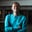Related Tags

php
communitycreator

# What are iterables in PHP?Zubair Idris Aweda

Iterables were introduced in PHP 7.4 as pseudo-types. These are not actual types and are only used to represent items that may be iterated on, like arrays or objects.

Any item said to be an iterable can be iterated using the foreach loop.

Iterables can be used to define a function parameter’s type to indicate that whatever input is being passed must be a set of values that may be iterated on.

### Examples

A function that multiplies all the elements of the array passed to it by 6 can be defined like this:

function times_six (iterable $numbers) { // Loop here }  Passing a non-iterable value to this function throws a TypeError. If you ever need to set a default for an expected iterable value, use an array (preferably empty) or null, like this: function times_six (iterable$numbers = [])
{
// Loop here
}


Iterables can also be used to define a function’s return type.

For example, the function times_six defined earlier can have an iterable return type, as it will return an array of products of six.

function times_six (iterable $numbers = []): iterable { // Loop here }  ### Code Let’s use the foreach loop to iterate over an array of numbers. <?php function times_six(iterable$numbers){
foreach($numbers as$number){
echo $number * 6; // Multiply each number in array by 6 echo "\n"; } } echo "Using Iterables in PHP:\n";$numbers = array(1, 2, 3, 4, 5); // original array
times_six(\$numbers);
?>
Iterables in PHP

RELATED TAGS

php
communitycreator

CONTRIBUTORZubair Idris Aweda
RELATED COURSES

View all Courses

Keep Exploring

Learn in-demand tech skills in half the time